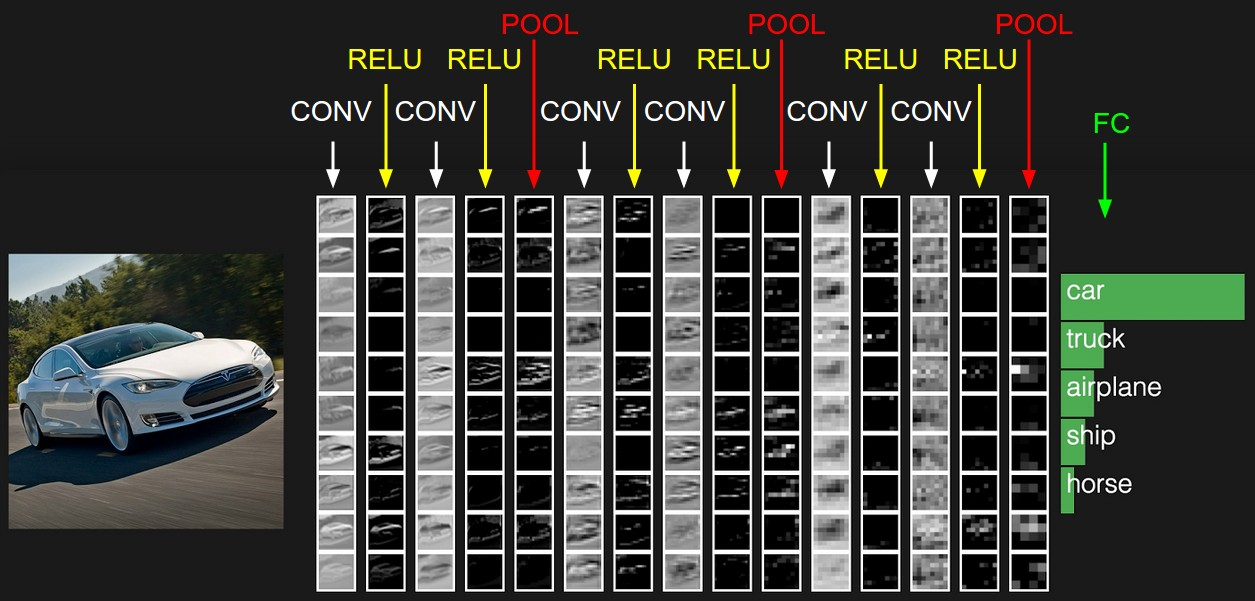# Convolution Neural Network

Here we basically follow the contents provided by CS231n Convolutional Neural Networks for Visual Recognition. A related video can be found in Youtube.

Another tutorial of TensorFlow on deep NN is provided by Chelsea Finn from Berkeley CS 294 course.

A simple implementation with Pytorch can be found here.

The major advantage of CNN is “exploiting the local spatial correlations present in images”.

## Architecture Overview

Note that, according to Convolutional Neural Networks for Visual Recognition, Stanford, CS231n “ConvNet architectures make the explicit assumption that the inputs are images”.

One reason that we need ConvNN for images is that regular NNs do not scale well to full images.

ConvNN is made up of layers, each layer transform a 3D volumn to another 3D volumn with differentiable functions. Three types of layers are used: Convolutional Layer, Pooling Layer, and Fully-Connected Layer (exactly as seen in regular Neural Networks)

(Figures come from Convolutional Neural Networks for Visual Recognition, Stanford, CS231n)

In this way, ConvNets transform the original image layer by layer from the original pixel values to the final class scores.

## Layers used to build ConvNets

In summary,

• A ConvNet architecture is in the simplest case a list of Layers that transform the image volume into an output volume (e.g. holding the class scores)
• There are a few distinct types of Layers (e.g. CONV/FC/RELU/POOL are by far the most popular)
• Each Layer accepts an input 3D volume and transforms it to an output 3D volume through a differentiable function
• Each Layer may or may not have parameters (e.g. CONV/FC do, RELU/POOL don’t)
• Each Layer may or may not have additional hyperparameters (e.g. CONV/FC/POOL do, RELU doesn’t)### Convolutional Layer

Convolutional layer is the key section of ConvNN. In convolutional layer, some filters (specific edges, colors…) are used to slides along width and height for all depth. For example, we use 12 filters with size 5*5*3 to on a 32*32*3 image, the output volumn will be 32*32*12 (we stack 12 2D volumns).

Each pixel is locally connected to the corresponding pixel in the input volumn.

Three hyperparameters control the size of the output volume: the depth, stride and zero-padding.

The depth corresponds to how many filters we would like to use in the convolution.

The stride is the step length we would like to move when we slides along width or height. 1 or 2 is commonly used as stride while 3 or more is rare in practice.

Zero-padding is a hyperparameter handling with border elements. Generally, zero padding of 1 is used to keep the output size same as input size.

### Pooling Layer

It is common to periodically insert a Pooling layer in-between successive Conv layers in a ConvNet architecture. Pooling can reduce the parameters in the ConvNN dramatically and hence avoid overfitting.

Generally, a 2*2 filter is used with stride 2 to do downsammpling. For a 2D volumn, only 25% content will be kept after pooling.

### Fully-connected Layer

Neurons in a fully connected layer have full connections to all activations in the previous layer, as seen in regular Neural Networks.

## ConvNet Architectures

### Layer Patterns

The most common ConvNet architecture follows the pattern:

INPUT -> [[CONV -> RELU]*N -> POOL?]*M -> [FC -> RELU]*K -> FC


where the * indicates repetition, and the POOL? indicates an optional pooling layer. Moreover, N >= 0 (and usually N <= 3), M >= 0, K >= 0 (and usually K < 3). For example, here are some common ConvNet architectures you may see that follow this pattern:

• INPUT -> FC, implements a linear classifier. Here N = M = K = 0.
• INPUT -> CONV -> RELU -> FC
• INPUT -> [CONV -> RELU -> POOL]*2 -> FC -> RELU -> FC. Here we see that there is a single CONV layer between every POOL layer.
• INPUT -> [CONV -> RELU -> CONV -> RELU -> POOL]*3 -> [FC -> RELU]*2 -> FC Here we see two CONV layers stacked before every POOL layer. This is generally a good idea for larger and deeper networks, because multiple stacked CONV layers can develop more complex features of the input volume before the destructive pooling operation.

However, you should rarely ever have to train a ConvNet from scratch or design one from scratch. Instead, find what is popular in ImageNet and download that pretrained model.

### Layer Sizing Patterns

This subsection remains to be built when I have more practice.

## Refs.

 Mnih, V., Kavukcuoglu, K., Silver, D., Rusu, A.A., Veness, J., Bellemare, M.G., Graves, A., Riedmiller, M., Fidjeland, A.K., Ostrovski, G. and Petersen, S., 2015. Human-level control through deep reinforcement learning. nature, 518(7540), pp.529-533.